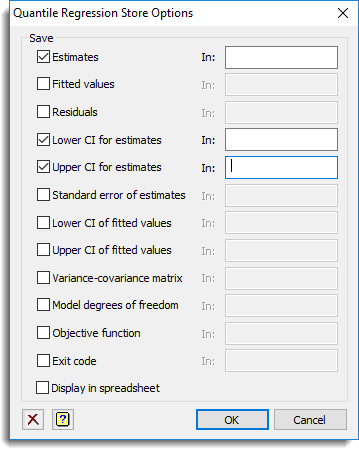1. Home
2. Quantile Regression Store Options

# Quantile Regression Store Options

This dialog lets you save results from a Quantile regression. These results are saved when an analysis is run and must be specified before running an analysis.

1. After selecting the appropriate boxes, type names for the data structures into the corresponding In: fields.## Save

The table below indicates the type of structures formed for each item. If more than one quantile has been specified then the structures formed will be a pointer or variate instead of a variate or scalar for a single quantile.

 Estimates Variate or pointer Estimated coefficients of model terms for each quantile. Fitted values Variate or pointer Fitted values from the regression for each quantile. Residuals Variate or pointer Residuals from regression for each quantile. Lower CI for estimates Variate or pointer Lower confidence limit of coefficients for each quantile (requires bootstrapping to be performed). Upper CI for estimates Variate or pointer Upper confidence limit of coefficients for each quantile (requires bootstrapping to be performed). Standard error of estimates Variate or pointer Standard errors of the estimated coefficients for each quantile (requires bootstrapping to be performed). Lower CI of fitted values Variate or pointer Lower confidence limit of fitted values for each quantile (requires bootstrapping to be performed). Lower CI of fitted values Variate or pointer Upper confidence limit of fitted values for each quantile (requires bootstrapping to be performed). Variance covariance matrix Symmetric matrix or pointer Variance-covariance matrix of estimates for each quantile (requires bootstrapping to be performed). Model degrees of freedom Scalar or variate Numbers of degrees of freedom fitted by the model. Objective function Scalar or variate Optimal values of the objective function SUM(e*(Q – (e > 0))) where e = Y – Xβ. Exit code Scalar Exit codes indicating whether the estimation was successful. A exit code of 0 indicates success, 1 that the model fitted but that there was no a unique optimum solution, and 2 that the simplex algorithm failed.

Select this to display the results in a new spreadsheet window.

## Action buttons

 OK Save the store settings and close the dialog. Cancel Close the dialog without making any changes.

## Action IconsClear Clear all fields and list boxes.Help Open the Help topic for this dialog.